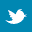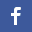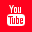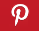Completely Solved C, C++ Programs Assignment.Quick Search Database Videos Tutorials Ebooks Forums FAQ Aboutus Household Industrial Manufacturing Service Shopping Transportation### C program to perform the different operations on a binary search tree

 Filed Under: C Assignments

Program Statement :
Write a C program to perform the following operations on a binary search tree :-
3. Inserting a given set of data recursively.
4. Implementing preorder, inorder and postorder traversals non-recursively.
5. Deleting any node with a specified data.

Theory :
A binary search tree (BST) T is a binary tree; either it is empty or each node in the tree contains an identifier and -
● All identifiers in the left subtree of T are less than the identifier in the root node T.
● All identifiers in the right subtree of T are greater than or equal to the identifier in the root node T.
● The left and right subtrees of T are also binary search trees.

We can perform following operations on a binary search tree :
● Inserting a node.
● Traversing the tree.
● Deleting a node.

 Inserting a node
The insertion operation is conceptually very simple. For inserting a new node we examine the root and recursively insert the new node to the left subtree if the new value is less than the root, or the right subtree if the new value is greater than or equal to the root.
 Traversing the tree
Common tree traversal algorithms i.e. Preorder, inorder and postorder traversals are also applicable for B.S.T without any alteration.
 Deleting a node
This operation is slightly more complicated than the previous two. Deletion of a node N depends on the number of its children. Hence three cases may arise :
1. Case 1 (N is the leaf node) : N is deleted by simply setting the pointer of N in PARENT(N) [denotes parent node of N] by NULL value.
2. Case 2 (N has exactly one child) : N is deleted by simply replacing the pointer of N in PARENT(N) by the pointer of only child of N.
3. Case 3 (N has two children) : N is deleted by first deleting SUCC(N) [denotes inorder successor of N] and then replacing the data content of in node N by the data content in node SUCC(N). Reset the left child of the parent of SUCC(N) by the right child of SUCC(N).

Algorithm :
&#61548; Insertion

/*This function inserts an element into the tree*/
Algo_insert(item)
{
/*Check if item already present in the tree*/
find(item,parent,location);
if(location!=NULL)
{
End Algorithm;
}
tmp=getnode();
tmp->info=item;
tmp->lchild=NULL;
tmp->rchild=NULL;
if(parent==NULL)
root=tmp;
else
if(item<parent->info)
parent->lchild=tmp;
else
parent->rchild=tmp;
}
/*End of Algo_insert*/

&#61548; Traversal

Preorder
/*Tree traversal in non-recursive preorder */
Algo_nrec_pre(struct node *ptr)
{
push(ptr);
while(top!=-1)
{

ptr=pop();
if(ptr!=NULL)
{
print(ptr->info);
push(ptr->rchild);
push(ptr->lchild);
}
}
}
/*End of Algo_nrec_pre*/

Inorder
/*Tree traversal in non-recursive inorder*/
Algo_nrec_in(struct node *ptr)
{
while(top!=-1OR ptr!=NULL)
{
if(ptr!=NULL)
{

push(ptr);
ptr=ptr->lchild;
}
else
{
ptr=pop();
print(ptr->info);
ptr=ptr->rchild;
}
}
}
/*End of Algo_nrec_in*/

Postorder
/*Tree traversal in non-recursive postorder*/
Algo_nrec_post(struct node *ptr)
{
push(NULL);
do
{
while(ptr!=NULL)
{

push(ptr);
flag[top]=1;
if(ptr->rchild!=NULL)
{
push(ptr->rchild);
flag[top]=-1;
}
ptr=ptr->lchild;
}
top_prev=top;
ptr=pop();
while(flag[top_prev]==1)
{
print(ptr->info);
top_prev=top;
ptr=pop();
}
}while(ptr!=NULL);
}
/*End of Algo_nrec_post*/

&#61548; Deletion
/*Case in which no child is present*/
Algo_case_a(struct node *par,struct node *loc )
{
if(par==NULL) /*item to be deleted is root node*/
root=NULL;
else
if(loc==par->lchild)
par->lchild=NULL;
else
par->rchild=NULL;
}
/*End of Algo_case_a*/

/*Case in which one child is present*/
Algo_case_b(struct node *par,struct node *loc)
{
/*Initialize child*/
if(loc->lchild!=NULL) /*item to be deleted has lchild */
child=loc->lchild;
else /*item to be deleted has rchild */
child=loc->rchild;

if(par==NULL ) /*Item to be deleted is root node*/
root=child;
else
if( loc==par->lchild) /*item is lchild of its parent*/
par->lchild=child;
else /*item is rchild of its parent*/
par->rchild=child;
}
/*End of Algo_case_b*/

/*Case in which two children are present*/
Algo_case_c(struct node *par,struct node *loc)
{
/*Find inorder successor and its parent*/
ptrsave=loc;
ptr=loc->rchild;
while(ptr->lchild!=NULL)
{
ptrsave=ptr;
ptr=ptr->lchild;
}
suc=ptr;
parsuc=ptrsave;

if(suc->lchild==NULL && suc->rchild==NULL)
case_a(parsuc,suc);
else
case_b(parsuc,suc);

if(par==NULL) /*if item to be deleted is root node */
root=suc;
else
if(loc==par->lchild)
par->lchild=suc;
else
par->rchild=suc;

suc->lchild=loc->lchild;
suc->rchild=loc->rchild;
}
/*End of Algo_case_c*/

Program listing :
/*C progam to perform the following operations on a binary search tree
*1.Inserting a given set of data recursively.
*2.Implementing preorder,inorder and postorder traversals non-recusively.
*3.Delete an element from tree.*/

# include <stdio.h>
# include <stdlib.h>
# include <alloc.h>
# define MAX 50/*Declaring maximum size of array used for stack and queue*/
struct node/*Declaring structure of a node*/
{
int info;
struct node *lchild;
struct node *rchild;
}*root;
struct node *stack[MAX];
int top=-1;/*Initializing top of stack*/
int front=-1,rear=-1;/*Initializing front and rear of queue*/
void find(int,struct node**,struct node**);
void main()
{
int choice,num,t,c,s,count=0,ch_t;
root=NULL;
void insert(int);
void del(int);
void nrec_pre(struct node*);
void nrec_in(struct node*);
void nrec_post(struct node*);
void display(struct node* ,int );
void level_trv(struct node* );
while(1)
{
printf("n1.Insertn");
printf("2.Traversaln");
printf("3.Deleten");
printf("4.Exitn");
scanf("%d",&choice);
switch(choice)
{
case 1:
printf("Enter the number to be inserted : ");
scanf("%d",&num);
insert(num);
count++;
break;
case 2:
printf("1.Inorder Traversaln");
printf("2.Preorder Traversaln");
printf("3.Postorder Traversaln");
printf("nEnter choice: ");
scanf("%d",&ch_t);
printf("n");
switch(ch_t)
{
case 1:
if(root==NULL)
{
printf("nTree is empty.n");
break ;
}
nrec_in(root);
break;
case 2:
if(root==NULL)
{
printf("nTree is empty.n");
break ;
}
nrec_pre(root);
break;
case 3:
if(root==NULL)
{
printf("nTree is empty.n");
break ;
}
nrec_post(root);
break;
default:
printf("nWrong choicen");
}
break;
case 3:
printf("Enter the number to be deleted : ");
scanf("%d",&num);
del(num);
break;
case 4: return;

default:
printf("nWrong choicen");
}
}
}

/*This function inserts an element into the tree*/
void insert(int item)
{
struct node *tmp,*parent,*location;
find(item,&parent,&location);
if(location!=NULL)
{
return;
}
tmp=(struct node *)malloc(sizeof(struct node));
tmp->info=item;
tmp->lchild=NULL;
tmp->rchild=NULL;
if(parent==NULL)
root=tmp;
else
if(item<parent->info)
parent->lchild=tmp;
else
parent->rchild=tmp;
}
/*Function to find the position of insertion */

void find(int item,struct node **par,struct node **loc)
{
struct node *ptr,*ptrsave;
if(root==NULL)/*Tree empty*/
{
*loc=NULL;
*par=NULL;
return;
}
if(item==root->info)/*Item is at root*/
{
*loc=root;
*par=NULL;
return;
}
/*Initialize ptr and ptrsave*/
if(item<root->info)
ptr=root->lchild;
else
ptr=root->rchild;
ptrsave=root;
while(ptr!=NULL)/*Until end is reached*/
{
if(item==ptr->info)
{ *loc=ptr;
*par=ptrsave;
return;
}
ptrsave=ptr;
if(item<ptr->info)
ptr=ptr->lchild;
else
ptr=ptr->rchild;
}
*par=ptrsave;
}
/*Case in which no child is prsent*/
void case_a(struct node *par,struct node *loc )
{
if(par==NULL) /*item to be deleted is root node*/
root=NULL;
else
if(loc==par->lchild)
par->lchild=NULL;
else
par->rchild=NULL;
}
/*Case in which one child is present*/
void case_b(struct node *par,struct node *loc)
{
struct node *child;
/*Initialize child*/
if(loc->lchild!=NULL) /*item to be deleted has lchild */
child=loc->lchild;
else /*item to be deleted has rchild */
child=loc->rchild;

if(par==NULL ) /*Item to be deleted is root node*/
root=child;
else
if( loc==par->lchild) /*item is lchild of its parent*/
par->lchild=child;
else /*item is rchild of its parent*/
par->rchild=child;
}
/*Case in which two children are present*/
void case_c(struct node *par,struct node *loc)
{
struct node *ptr,*ptrsave,*suc,*parsuc;
/*Find inorder successor and its parent*/
ptrsave=loc;
ptr=loc->rchild;
while(ptr->lchild!=NULL)
{
ptrsave=ptr;
ptr=ptr->lchild;
}
suc=ptr;
parsuc=ptrsave;
if(suc->lchild==NULL && suc->rchild==NULL)
case_a(parsuc,suc);
else
case_b(parsuc,suc);
if(par==NULL) /*if item to be deleted is root node */
root=suc;
else
if(loc==par->lchild)
par->lchild=suc;
else
par->rchild=suc;
suc->lchild=loc->lchild;
suc->rchild=loc->rchild;
}
/*This function deletes an element from tree*/
void del(int item)
{
struct node *parent,*location;
if(root==NULL)
{
printf("Tree empty");
return;
}
find(item,&parent,&location);
if(location==NULL)
{
printf("Item not present in tree");
return;
}
if(location->lchild==NULL && location->rchild==NULL)
case_a(parent,location);
if(location->lchild!=NULL && location->rchild==NULL)
case_b(parent,location);
if(location->lchild==NULL && location->rchild!=NULL)
case_b(parent,location);
if(location->lchild!=NULL && location->rchild!=NULL)
case_c(parent,location);
free(location);
}
/*Tree traversal in non-recursive preorder */
void nrec_pre(struct node *ptr)
{
void push(struct node*);
struct node* pop();
push(ptr);
while(top!=-1)
{
ptr=pop();
if(ptr!=NULL)
{
printf("%d ",ptr->info);
push(ptr->rchild);
push(ptr->lchild);
}
}
}
/*Tree traversal in non-recursive inorder*/
void nrec_in(struct node *ptr)
{
void push(struct node*);
struct node* pop();
while(top!=-1||ptr!=NULL)
{
if(ptr!=NULL)
{
push(ptr);
ptr=ptr->lchild;
}
else
{
ptr=pop();
printf("%d ",ptr->info);
ptr=ptr->rchild;
}
}
printf("nn");
}
/*Tree traversal in non-recursive postorder*/
void nrec_post(struct node *ptr)
{
void push(struct node*);
struct node* pop();
int flag[MAX];
int top_prev;
push(NULL);
do
{
while(ptr!=NULL)
{
push(ptr);
flag[top]=1;
if(ptr->rchild!=NULL)
{
push(ptr->rchild);
flag[top]=-1;
}
ptr=ptr->lchild;
}
top_prev=top;
ptr=pop();
while(flag[top_prev]==1)
{
printf("%d ",ptr->info);
top_prev=top;
ptr=pop();
}
}while(ptr!=NULL);
printf("nn");
}
/*This function pushes an element into strack*/
void push(struct node *node)
{
if(top > MAX)
{
printf("Stack overflown");
return;
}
else
{
top=top+1;
stack[top] = node;
}
}
/*This function pops an element from stack*/
struct node *pop()
{
if (top == -1 )
{
printf("Stack underflow n");
return;
}
else
return (stack[top--]);
}

Output :

1.Insert
2.Traversal
3.Delete
4.Exit

Enter the number to be inserted : 22

1.Insert
2.Traversal
3.Delete
4.Exit

Enter the number to be inserted : 56

1.Insert
2.Traversal
3.Delete
4.Exit

Enter the number to be inserted : -98

1.Insert
2.Traversal
3.Delete
4.Exit

Enter the number to be inserted : -76

1.Insert
2.Traversal
3.Delete
4.Exit

Enter the number to be inserted : 1222

1.Insert
2.Traversal
3.Delete
4.Exit

1.Inorder Traversal
2.Preorder Traversal
3.Postorder Traversal

Enter choice: 1

-98 -76 22 56 1222

1.Insert
2.Traversal
3.Delete
4.Exit

1.Inorder Traversal
2.Preorder Traversal
3.Postorder Traversal

Enter choice: 2

22 -98 -76 56 1222

1.Insert
2.Traversal
3.Delete
4.Exit

1.Inorder Traversal
2.Preorder Traversal
3.Postorder Traversal

Enter choice: 3

-76 -98 1222 56 22

1.Insert
2.Traversal
3.Delete
4.Exit

Enter the number to be deleted : 1222

1.Insert
2.Traversal
3.Delete
4.Exit

1.Inorder Traversal
2.Preorder Traversal
3.Postorder Traversal

Enter choice: 1

-98 -76 22 56

1.Insert
2.Traversal
3.Delete
4.Exit

Enter the number to be deleted : 89
Item not present in tree

1.Insert
2.Traversal
3.Delete
4.Exit

1.Inorder Traversal
2.Preorder Traversal
3.Postorder Traversal

Enter choice: 2

22 -98 -76 56

1.Insert
2.Traversal
3.Delete
4.Exit

Discussions :
 Binary search trees are a fundamental data structure used to construct more abstract data structures such as sets, multi sets, and associative arrays.
 Inorder traversal on a B.S.T give the sorted order of data in ascending order. To sort a set of data a B.S.T can be built with those data and then inorder traversal can be applied. This method is known as binary sort and this is why a B.S.T is also called a binary sorted tree.
 This operation requires O(log n) time in the average case, but needs O(n) time in the worst-case, when the unbalanced tree resembles a linked list (degenerate tree).
 There are many types of binary search trees. AVL trees and red-black trees are both forms of self-balancing binary search trees. A splay tree is a binary search tree that automatically moves frequently accessed elements nearer to the root. In a treap ("tree heap"), each node also holds a priority and the parent node has higher priority than its children.
 Two other titles describing binary search trees are that of a complete and degenerate tree.Back to main directory:Software Practical